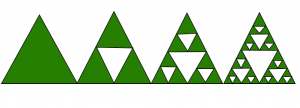GeeksforGeeks App
Open AppBrowser
Continue

# Sierpinski triangle

Sierpinski triangle is a fractal and attractive fixed set with the overall shape of an equilateral triangle. It subdivides recursively into smaller triangles.Examples :

```Input : n = 4
Output :
*
* *
*   *
* * * *

Input : n = 8
Output :
*
* *
*   *
* * * *
*       *
* *     * *
*   *   *   *
* * * * * * * * ```

Approach :

Sierpinski Triangle will be constructed from an equilateral triangle by repeated removal of triangular subsets.
Steps for Construction :
1 . Take any equilateral triangle .
2 . Divide it into 4 smaller congruent triangle and remove the central triangle .
3 . Repeat step 2 for each of the remaining smaller triangles forever.

Below is the program to implement Sierpinski triangle

## C++

 `// C++ program to print sierpinski triangle.``#include ``using` `namespace` `std;`` ` `void` `printSierpinski(``int` `n)``{``    ``for` `(``int` `y = n - 1; y >= 0; y--) {`` ` `        ``// printing space till``        ``// the value of y``        ``for` `(``int` `i = 0; i < y; i++) {``            ``cout<<``" "``;``        ``}`` ` `        ``// printing '*'``        ``for` `(``int` `x = 0; x + y < n; x++) {`` ` `        ``// printing '*' at the appropriate position``        ``// is done by the and value of x and y``        ``// wherever value is 0 we have printed '*'``        ``if``(x & y)``            ``cout<<``" "``<<``" "``;``        ``else``            ``cout<<``"* "``;``        ``}`` ` `        ``cout<

## Java

 `// Java program to print ``// sierpinski triangle.``import` `java.util.*;``import` `java.io.*;`` ` `class` `GFG ``{``    ``static` `void` `printSierpinski(``int` `n)``    ``{``        ``for` `(``int` `y = n - ``1``; y >= ``0``; y--) {`` ` `            ``// printing space till``            ``// the value of y``            ``for` `(``int` `i = ``0``; i < y; i++) {``                ``System.out.print(``" "``);``            ``}`` ` `            ``// printing '*'``            ``for` `(``int` `x = ``0``; x + y < n; x++) {`` ` `                ``// printing '*' at the appropriate``                ``// position is done by the and ``                ``// value of x and y wherever value``                ``// is 0 we have printed '*'``                ``if` `((x & y) != ``0``)``                    ``System.out.print(``" "``                                    ``+ ``" "``);``                ``else``                    ``System.out.print(``"* "``);``            ``}`` ` `            ``System.out.print(``"\n"``);``        ``}``    ``}`` ` `    ``// Driver code``    ``public` `static` `void` `main(String args[])``    ``{``        ``int` `n = ``16``;`` ` `        ``// Function calling``        ``printSierpinski(n);``    ``}``}`` ` `// This code is contributed by Sahil_Bansall`

## Python3

 `# Python 3 program to print ``# sierpinski triangle.`` ` `def` `printSierpinski( n) :``     ` `    ``y ``=` `n ``-` `1``    ``while``(y >``=` `0``) :``         ` `        ``# printing space till``        ``# the value of y``        ``i ``=` `0``        ``while``(i < y ):``            ``print``(``" "``,end``=``"")``            ``i ``=` `i ``+` `1`` ` `        ``# printing '*'``        ``x ``=` `0``        ``while``(x ``+` `y < n ):`` ` `            ``# printing '*' at the appropriate``            ``# position is done by the and ``            ``# value of x and y wherever value``            ``# is 0 we have printed '*'``            ``if` `((x & y) !``=` `0``) :``                ``print``(``" "``, end ``=` `" "``)``            ``else` `:``                ``print``(``"* "``, end ``=` `"")``            ``x ``=``x ``+` `1``         ` `        ``print``()``        ``y ``=` `y ``-` `1``         ` `# Driver code``n ``=` `16`` ` `# Function calling``printSierpinski(n)`` ` ` ` `# This code is contributed by Nikita Tiwari.`

## C#

 `// C# program to print``// sierpinski triangle.``using` `System;`` ` `class` `GFG {``    ``static` `void` `printSierpinski(``int` `n)``    ``{``        ``for` `(``int` `y = n - 1; y >= 0; y--) {`` ` `            ``// printing space till``            ``// the value of y``            ``for` `(``int` `i = 0; i < y; i++) {``                ``Console.Write(``" "``);``            ``}`` ` `            ``// printing '*'``            ``for` `(``int` `x = 0; x + y < n; x++) {`` ` `                ``// printing '*' at the appropriate``                ``// position is done by the and``                ``// value of x and y wherever value``                ``// is 0 we have printed '*'``                ``if` `((x & y) != 0)``                    ``Console.Write(``" "` `+ ``" "``);``                ``else``                    ``Console.Write(``"* "``);``            ``}`` ` `            ``Console.WriteLine();``        ``}``    ``}`` ` `    ``// Driver code``    ``public` `static` `void` `Main()``    ``{``        ``int` `n = 16;`` ` `        ``// Function calling``        ``printSierpinski(n);``    ``}``}`` ` `// This code is contributed by vt_m`

## PHP

 `= 0; ``\$y``--) ``    ``{`` ` `        ``// printing space till``        ``// the value of y``        ``for` `(``\$i` `= 0; ``\$i` `< ``\$y``; ``\$i``++)``        ``{``            ``echo` `" "``;``        ``}`` ` `        ``// printing '*'``        ``for` `(``\$x` `= 0; ``\$x` `+ ``\$y` `< ``\$n``; ``\$x``++) ``        ``{`` ` `        ``// printing '*' at the appropriate ``        ``// position is done by the and value ``        ``// of x and y wherever value is 0 we ``        ``// have printed '*'``        ``if``(``\$x` `& ``\$y``)``            ``echo``"  "``;``        ``else``            ``echo``"* "``;``        ``}`` ` `        ``echo` `"\n"``;``    ``}``}`` ` `// Driver code``\$n` `= 16;``printSierpinski(``\$n``);`` ` `// This code is contributed by Mithun Kumar``?>`

## Javascript

 ``

Output :

```               *
* *
*   *
* * * *
*       *
* *     * *
*   *   *   *
* * * * * * * *
*               *
* *             * *
*   *           *   *
* * * *         * * * *
*       *       *       *
* *     * *     * *     * *
*   *   *   *   *   *   *   *
* * * * * * * * * * * * * * * * ```

Time complexity: O(n2
Auxiliary space: O(1)

References : Wiki

My Personal Notes arrow_drop_up